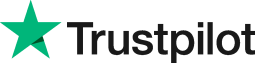vlookup vs hlookup
• Home
• Courses
• Promotions
• Schedule
• Formats
• Our Clients

Forum home » Topics » vlookup vs hlookup

# vlookup vs hlookup

Lookup functions let you search a table of records and return a corresponding value when Excel finds a record that matches.

For example a user might have a table containing 100 currencies and their latest exchange rates. When the user enters a currency code into the lookup Excel searches for a matching code in the table. When it finds a match Excel returns the correct exchange rate for that currency.

There are two types of lookup - Vertical (Vlookup) or Horizontal (Hlookup) - depending on how your table of data is laid out in the sheet.

Lookups can search tables of data in other sheets or workbooks.

The syntax of a lookup is as follows:

VLOOKUP(Table of data, column in the table that contains the result, approximate match TRUE or exact match FALSE)

Bear in mind that that the first column in your table of data is the column that the lookup will search. If you are using an approximate match then your table must be sorted in ascending order, based on this column.

# Related forum posts:

## HLook up formulas

could you please let me know how it works? Thanks

see above

## HLOOKUP vs VLOOKUP

HLOOKUP: What's the difference between this and VLOOKUP?

## Difference between Look ups

What's the difference between V and Hlookups

## HLOOKUP

How do i do a hlookup?

# Related articles

## How To Use Vlookup In Excel

Have you wanted to find out how to use Excel's vlookup function? This function is used to extract data from a range of cells and display the extracted data in a different cell. This article describes the elements which make up vlookup and then explains what vlookup does and describes how to use this function in clear easy to follow steps.

 Welcome. Please choose your application (eg. Excel) and then post your question. Our Microsoft Qualified trainers will then respond within 24 hours (working days). Frequently Asked Questions What does 'Resolved' mean? Any suggestions, questions or comments? Please post in the Improve the forum thread.TrustScore 4.8 / 5       1238 reviews